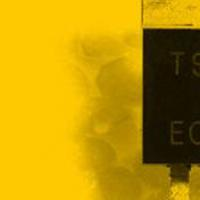## K-Means clustering in OpenCV

K-Means is an algorithm to detect clusters in a given set of points. It does this without you supervising or correcting the results. It works with any number of dimensions as well (that is, it works on a plane, 3D space, 4D space and any other finite dimensional spaces). And OpenCV comes with this algorithm built right into it!

## K-means with OpenCV's C++ interface

The function you need to call to execute the algorithm is:

```double kmeans(const Mat& samples,
int clusterCount,
Mat& labels,
TermCriteria termcrit,
int attempts,
int flags,
Mat* centers)
```

This function is in the cv namespace. So you can use it by cv::kmeans or by simply including the cv namespace. If you know how K-means works, the parameters should be self explanatory.

### Parameters

• `samples`: (input) The actual data points that you need to cluster. It should contain exactly one point per row. That is, if you have 50 points in a 2D plane, then you should have a matrix with 50 rows and 2 columns.
• `clusterCount`: (input) The number of clusters in the data points.
• `labels`: (output) Returns the cluster each point belongs to. It can also be used to indicate the initial guess for each point.
• `termcrit`: (input) This is an iterative algorithm. So you need to specify the termination criteria (number of iterations & desired accuracy)
• `attempts`: (input) The number of times the algorithm is run with different center placements
• `flags`: (input) Possible values include:
• `KMEANS_RANDOM_CENTER`: Centers are generated randomly
• `KMEANS_PP_CENTER`: Uses the kmeans++ center initialization
• `KMEANS_USE_INITIAL_LABELS`: The first iteration uses the supplied labels to calculate centers. Later iterations use random or semi-random centers (use the above two flags for that).
• `centers`: (output) This matrix holds the center of each cluster.

### Returns

The function returns the compactness of the final clustering. What is compactness? It's a measure of how good the labeling was done. The smaller the better.

When attempts is 1, the value returned is the compactness of the only iteration that happened. If attempts is more than 1, the final labeling returned is the one with the least compactness.

## K-means with OpenCV's C interface

The C equivalent of the k-means function is:

```int cvKMeans2(const CvArr* samples,
int nclusters,
CvArr* labels,
CvTermCriteria termcrit,
int attempts=1,
CvRNG* rng=0,
int flags=0,
CvArr* centers=0,
double* compactness=0)
```

The parameters are similar to the C++ interface.

### Parameters

• `samples`: (input) The actual data points that you need to cluster. It should contain exactly one point per row.
• `nclusters`: (input) The number of clusters in the data points.
• `labels`: (output) Returns the cluster each point belongs to. It can also be used to indicate the initial guess for each point.
• `termcrit`: (input) This is an iterative algorithm. So you need to specify the termination criteria (number of iterations & desired accuracy)
• `attempts`: (input) The number of times the algorithm is run with different center placements
• `rng`: (input) A random number generate used to generate the initial guess. Puts you in total control of what's happening.
• `flags`: (input) Possible values include:
• `0`: (the number 0) Centers are generated randomly
• `KMEANS_USE_INITIAL_LABELS`: The first iteration uses the supplied labels to calculate centers. Later iterations use random or semi-random centers (use the above two flags for that).
• `centers`: (output) This matrix holds the center of each cluster.
• `compactness`: (output) Holds the compactness of the best labeling scheme.

If you're still using the C interface, I highly recommend you shift to the more intuitive and no-more-tears C++ interface!

## Summary

You got to know how to run K-means without writing any code! You got to know about the C++ and C functions that you can use to execute K-Means on your data sets.

#### Related posts#### 78xx voltage regulator#### 7 unique neural network architectures#### Community Core Vision: An app for simple vision stuff

Utkarsh Sinha created AI Shack in 2010 and has since been working on computer vision and related fields. He is currently at Microsoft working on computer vision.